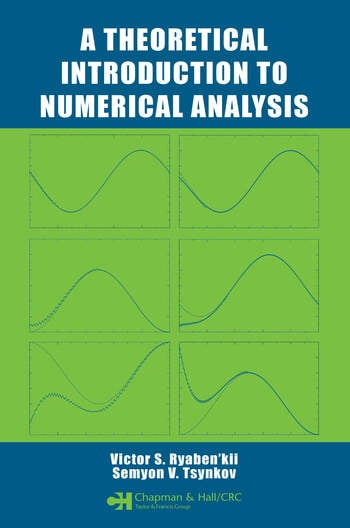# A Theoretical Introduction to Numerical Analysis

## 1st Edition

Victor S. Ryaben'kii, Semyon V. Tsynkov

Chapman and Hall/CRC
Published November 2, 2006
Textbook - 552 Pages - 50 B/W Illustrations
ISBN 9781584886075 - CAT# C6072

For Instructors Request Inspection Copy

USD\$110.00

Currently out of stock
FREE Standard Shipping!

## Preview

### Summary

A Theoretical Introduction to Numerical Analysis presents the general methodology and principles of numerical analysis, illustrating these concepts using numerical methods from real analysis, linear algebra, and differential equations. The book focuses on how to efficiently represent mathematical models for computer-based study.

An accessible yet rigorous mathematical introduction, this book provides a pedagogical account of the fundamentals of numerical analysis. The authors thoroughly explain basic concepts, such as discretization, error, efficiency, complexity, numerical stability, consistency, and convergence. The text also addresses more complex topics like intrinsic error limits and the effect of smoothness on the accuracy of approximation in the context of Chebyshev interpolation, Gaussian quadratures, and spectral methods for differential equations. Another advanced subject discussed, the method of difference potentials, employs discrete analogues of Calderon’s potentials and boundary projection operators. The authors often delineate various techniques through exercises that require further theoretical study or computer implementation.

By lucidly presenting the central mathematical concepts of numerical methods, A Theoretical Introduction to Numerical Analysis provides a foundational link to more specialized computational work in fluid dynamics, acoustics, and electromagnetism.

#### Instructors

We provide complimentary e-inspection copies of primary textbooks to instructors considering our books for course adoption.